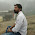# HackerRank solution for Functions In C++

Problem:- Hacker Rank Solution Program In C++ For "Functions " or Hacker Rank Solution Program In C++ For Functions or Functions solution hacker rank or Hacker rank solution for c++ domain or Hacker rank solution for Functions subdomain or Functions solution in c++ of hacker rank or introduction solutions hacker rank.

Check This:- Geeksforgeeks solution for School, Basic, Easy, Medium, Hard in C++.

Logic:- So function solution in c++ is very simple and easy t understand, first we have to understand what is a function? After that, we can use the function. Now come to the point hare we have to pass the value in a function, we can do this bypass by value and pass by reference(basically we passed the address of a variable), but here we are passing the value in the function. After the passing the value putting some condition in function and returning the output form function. for better understand let's see program step by step.

What is a function?

A function is a group of statements that together perform a task. Most important every C/C++ program has at least one function same Java program has one class at least.Main() is the function in C/C++.

Syntax of Function

return_type function_name( parameter list )
{
statement.......1 //a body of the function
statement.......2
statement.......3
.
.
.
statement.......n
}

Explanation:- First function declaration(optional if you are define function above main() function). After that taking a user input in this case 4 value is taking and pass the values in function then the function will return the greatest value for four value by some statement and condition.

Passing the value in function

max=max_of_four(a,b,c,d);

Optional Hint:- No need to use a huge number of the header file if you want to use only one header file you can use the Master Header file. Master header in C++ is #include<bits/stdc++.h> after adding this header file no need to add any other header file.

Solution:-

Method 1:- Here I have written return statement after each output for the understanding purpose, that is not compulsory to write return after each and every statement you can see method 2 full solution with one return.

#include <iostream>
#include <cstdio>
using namespace std;

int max_of_four(int a, int b, int c, int d)
{
if(a>b&&a>c&&a>d)
{
cout<<a;
return(a);
}
else if(b>a&&b>c&&b>d)
{
cout<<b;
return(b);
}
else if(c>b&&c>a&&c>d)
{
cout<<c;
return(c);
}
else
{
cout<<d;
return(d);
}
}

int main()
{
int a, b, c, d,max;
cin>>a>>b>>c>>d;
max=max_of_four(a,b,c,d);
}

Method 2:- No need to write return after each and every output just write return after all the cases and output.

#include <iostream>
#include <cstdio>
using namespace std;

int max_of_four(int a, int b, int c, int d)
{

if(a>b&&a>c&&a>d)
{
cout<<a;
}
else if(b>a&&b>c&&b>d)
{
cout<<b;
}
else if(c>b&&c>a&&c>d)
{
cout<<c;
}
else
{
cout<<d;
}
return 0;
}

int main()
{
int a, b, c, d,max;
cin>>a>>b>>c>>d;
max=max_of_four(a,b,c,d);
}

Output:-

1. Output in Hacker Rank Editor

2. Output in Local Editor

You May Like This:-

1.2.1.